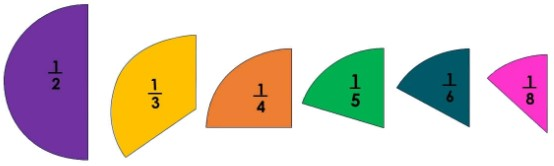# Least to Greatest Calculator (Ordering Fractions)

Enter the fractions, integers, percent, or decimal values separated by comma. Hit the Calculate button to arrange the given data in an order using ordering fractions calculator.

Give Us Feedback

## Least to Greatest Calculator

Least to greatest calculator is an online tool that can be used to order fractions from least to greatest (ascending order) and greatest to least (descending order) as well. This number sorter also tells us the total number of fractions and the least common denominator as well. By using this tool, you can order:

Moreover, the greatest to least calculator converts mixed numbers to fractions and fractions to decimals.

## How to order fractions?There are two methods to order fractions. We can arrange them by converting all fractions to decimals and then comparing them. In the second method, we arrange fractions by taking least common denominator (LCD).

Here we will discuss the fractions to decimal method to order fractions without using a number organizer.

Example:

Arrange the following fractions in ascending and descending order.

2/4, 5/2, 3/7, 9/4, 6/5

Solution:

Step 1: Convert all fractions to decimals to put these numbers in order.

2/4 = 0.5

5/2 = 2.5

3/7 = 0.42

9/4 = 2.25

6/5 = 1.2

Step 2: Compare all decimal values and arrange them accordingly.

Ascending Order = 0.42, 0.5, 1.2, 2.25, 2.5

Write the corresponding fractions in the same order.

Ascending Order = 3/7, 2/4, 6/5, 9/4, 5/2

Descending Order = 2.5, 2.25, 1.2, 0.5, 0.42

Place the corresponding fractions.

Descending Order = 5/2, 9/4, 6/5, 2/4, 3/7

### Math Tools